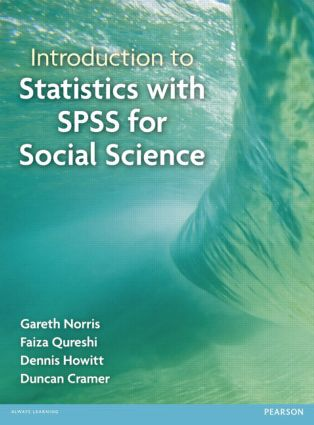Introduction to Statistics with SPSS for Social Science

1st Edition

Routledge

496 pages

Purchasing Options:\$ = USD
Paperback: 9781408237595
pub: 2012-05-31
SAVE ~\$12.79
\$63.95
\$51.16
x
Hardback: 9781138459007
pub: 2017-07-28
SAVE ~\$41.00
\$205.00
\$164.00
x
eBook (VitalSource) : 9781315833422
pub: 2014-09-19
from \$31.98

FREE Standard Shipping!

Description

This is a complete guide to statistics and SPSS for social science students. Statistics with SPSS for Social Science provides a step-by-step explanation of all the important statistical concepts, tests and procedures. It is also a guide to getting started with SPSS, and includes screenshots to illustrate explanations. With examples specific to social sciences, this text is essential for any student in this area.

Part One – Descriptive Statistics.

• Chapter 1 – Why you need statistics: types of data
• Chapter 2 – Describing variables: Tables and diagrams
• Chapter 3 – Describing variables numerically: averages, variation and spread
• Chapter 4 – Shapes of distributions of scores
• Chapter 5 - Standard deviation, z-scores and standard error: the standard unit of measurement in statistics
• Chapter 6 – Relationships between two or more variables: diagrams and tables
• Chapter 7 – Correlation coefficients: Pearson correlation and Spearman’s rho
• Chapter 8 – Regression and standard error

Part Two: Comparing Two or More Variables and the Analysis of Variance.

• Chapter 9 - The analysis of a questionnaire/survey project
• Chapter 10 – The related t-test: Comparing two samples of correlated/related scores
• Chapter 11 – the unrelated t-test: comparing two samples of unrelated/uncorrelated scores
• Chapter 12 – Chi-square: Differences between samples of frequency data

Part Three: Introduction to Analysis of Variance

• Chapter 13 – Analysis of variance (ANOVA): introduction to one-way unrelated or uncorrelated ANOVA
• Chapter 14 – Two way analysis of variance for unrelated/uncorrelated scores: two studies for the price of one?
• Chapter 15 – Analysis of covariance (ANCOVA): controlling for additional variables
• Chapter 16 – Multivariate analysis of variance (MANOVA)

Part Four: More advanced correlational statistics and techniques

• Chapter 17 - Partial correlation: spurious correlation, third or confounding variables (control variables), suppressor variables
• Chapter 18 – Factor analysis: simplifying complex data
• Chapter 19 – Multiple regression and multiple correlation
• Chapter 20 – Multinomial logistic regression: Distinguishing between several different categories or groups
• Chapter 21 - Bionomial logistic regression
• Chapter 22 - Log-linear methods: The analysis of complex contingency tables